Function Repository Resource:

# ExtendedGroebnerBasis

Compute a Groebner basis and a conversion matrix from the input polynomials to the basis

Contributed by: Daniel Lichtblau
 ResourceFunction["ExtendedGroebnerBasis"][polys,vars] gives the Groebner basis 𝒢 for the polynomial list polys along with a polynomial conversion matrix ℳ satisfying 𝒢=ℳ·polys.

## Details and Options

ResourceFunction["ExtendedGroebnerBasis"] takes the same optional arguments and options as GroebnerBasis.

## Examples

### Basic Examples (5)

Create a set of polynomials:

 In:=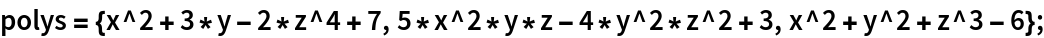First compute its Groebner basis:

 In:=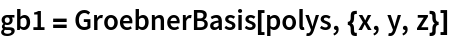Out=Compute the extended Groebner basis:

 In:=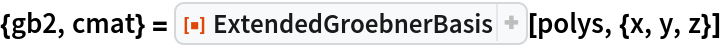Out=Check that the bases agree up to a constant factor:

 In:=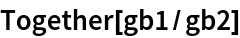Out=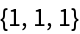Check the conversion matrix identity:

 In:=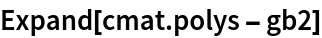Out=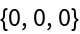### Scope (3)

Here is a larger example:

 In:=Out=In:=Out=Check that the bases agree up to constant factors (they are not identical in this instance):

 In:=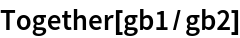Out=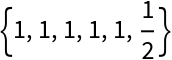Check the conversion matrix identity:

 In:=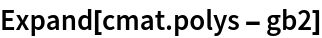Out=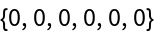### Properties and Relations (7)

The Groebner basis of a pair of univariate polynomials is their greatest common divisor, and similarly the extended Groebner basis gives the extended GCD. Create a pair of polynomials with a known common divisor poly1:

 In:=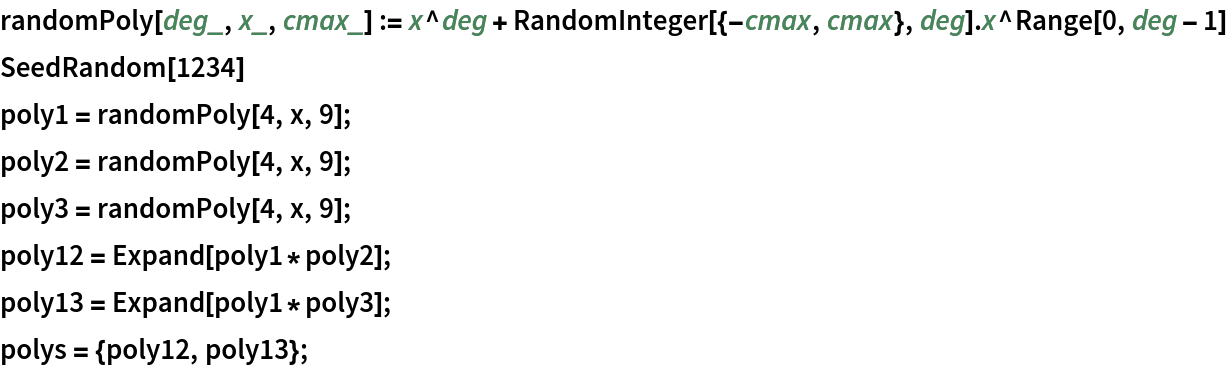Compute the extended GCD:

 In:=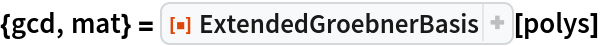Out=Check that the single polynomial in the Groebner basis is a GCD (a constant multiple of poly1):

 In:=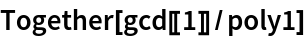Out=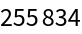Check the Bezout relation for the extended GCD:

 In:=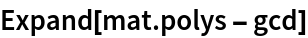Out=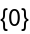Compare to PolynomialExtendedGCD:

 In:=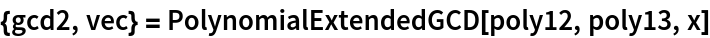Out=Again check the Bezout relation:

 In:=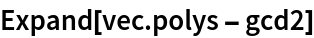Out=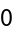Now check that these two computations agree up to a constant factor:

 In:=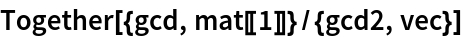Out=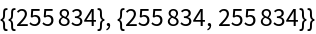### Possible Issues (1)

ExtendedGroebnerBasis can be much slower that GroebnerBasis for the same input:

 In:=Out=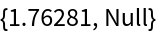In:=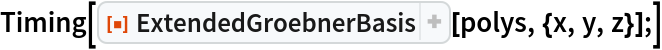Out=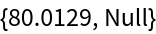## Version History

• 1.0.0 – 27 September 2019